• Call Now

1800-102-2727•

Type of Waves,Terms related to Waves , Practice Problems , FAQs

Have you thrown the stone in a water pool in your childhood? You have definitely seen a curve shape on the surface of water (As shown in figure). If a toy duck is placed in the path of this curve shape, the duck will start oscillating about their mean potion. Why is it happening so?This is due to Wave, when a stone is thrown in water energy is transferring to water, Due to elastic and inertial properties of matter this energy is propagating and reaches to the place of the Duck and it will start oscillating.Table of content

• What is Wave?
• Classification of waves
• Properties of wave
• Practice problems
• FAQs

## What is Wave?

Wave is a disturbance which carries energy and transfers from one place to another place.It can be in the form of elastic deformation, variation of pressure, electric field or magnetic field intensity etc. A general wave is shown in figure.## Classification of waves

Based on necessity of medium

1. Mechanical Waves

Wave which needs medium to propagate is called a mechanical wave. The speed of a mechanical wave depends on the elastic and inertial properties of the medium.

Example- Wave on a string, Sound wave, etc2. Non-mechanical Waves

Waves which do not need any medium to propagate and can easily travel through vacuum are termed as Non-mechanical waves. The speed of these waves in vacuum is a universal constant that is 299792458 m/s .

Example-Electromagnetic waves, Gravitational Waves.• Based on direction of particle motion

1. Transverse wave

Waves in which particles of medium moves perpendicular to the direction of propagation of waves known as Transverse waves.

Example- Wave on string, Water wave, S-type earthquake wave, Motion of spring shown in figure.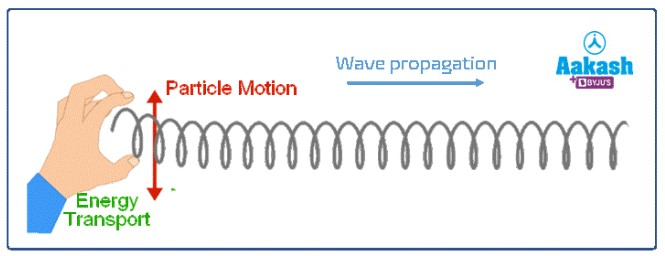2. Longitudinal wave

Waves in which the motion of a particle is along the direction of propagation of waves is known as longitudinal waves.

Example- Sound wave, P-type earthquake wave, Motion of spring shown in figure.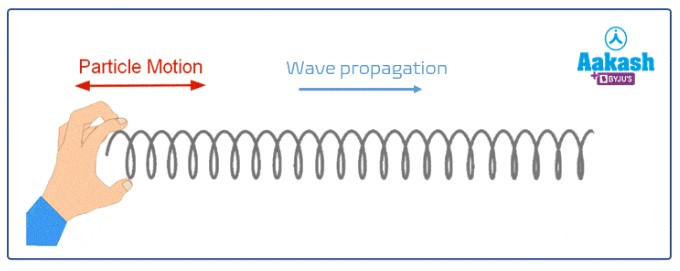• Based on propagation

1-Dimensional wave

Wave on string is an example of 1-Dimensional wave.2-Dimensional wave

Water wave is an example of a 2-Dimensional wave.3-Dimensional wave

Sound Wave is an example of a 3-Dimensional wave.## Properties of Wave

1. Amplitude- The maximum displacement of an oscillating particle from mean position is amplitude. Wave transfers the energy and Amplitude is directly related to energy. Intensity of any wave is directly proportional to square of amplitude.

2. Wavelength-The distance between two crests of successive cycles is known as Wavelength it is denoted by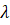,since it is length, its SI unit is (m).

3. Time period-Timeperiod of a wave is the time taken by the particle of medium to complete one oscillation denoted by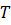. It is measured in seconds.

4. Frequency- Frequency of wave is the number of oscillations of a particle in a certain time. It is denoted by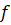measured in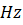which is equal to 1 oscillation per second.

Period and frequency are reciprocal of each other,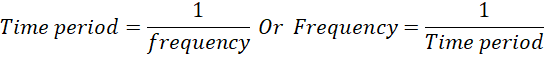5. Speed-speed of wave is actually the measurement of the rate of propagation of energy in the direction of wave propagation. Speed of the wave does not depend on the source, it depends on the inertial and elastic properties of the medium. For a medium speed of wave is constant.

Ifis the wavelength,is the time period andis the frequency of wave of a wave the speed of wave is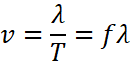## Practice problems

Que: Find the frequency of a wave if its time period is 0.1 s .

Ans: As you know the relation between time period and frequency is,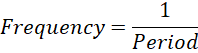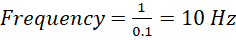.

Que: A wave has wavelength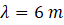and and time period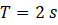Find the velocity of the wave?

Ans:Velocity of wave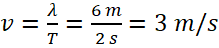.

Que: Consider a string wave as shown in figure.Then compute the following in both (assume wave crosses the distance in one second).1. Wavelength,
2. Frequency
3. Velocity

Ans:

Que: Average range of frequency at which human can hear the sound is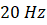to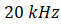. Calculate the wavelength of sound in thess limit.(assume the speed of sound in air is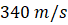).

Ans: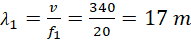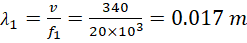Hence, the audible wavelength region is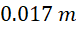to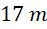.

## FAQs

Que: mechanical wave velocity depends on which property of medium?

Ans: wave velocity depends on inertial and elastic properties of the medium.

Que: What is the relation between Time period and frequency?

Ans: Time period is inversely proportional to frequency,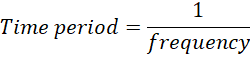Que: In which wave particle of moves perpendicular to the propagation of wave

Ans: In transverse waves, particles move perpendicular to wave motion.

Que: What are the non-mechanical waves?

Ans: waves which do not require medium to propagate are termed as non-mechanical waves. Example EM waves.Talk to our expert
Resend OTP Timer =
By submitting up, I agree to receive all the Whatsapp communication on my registered number and Aakash terms and conditions and privacy policy